# Trading Scenario: Margin Call Level at 100% and No Separate Stop Out LevelSebastian TR.Partner Center Find a Broker

Let’s now take all the margin jargon you’ve learned from the previous lessons and apply them by looking at trading scenarios with different Margin Call and Stop Out Levels.

Different retail forex brokers and CFD providers have different margin call policies. Some only operate only with Margin Calls, while others define separate Margin Call and Stop Out Levels.

In this lesson, we will go through a real-life trading scenario where you are using a broker that only operates with a Margin Call.

The broker defines its Margin Call Level at 100% and has no separate Stop Out Level.

What happens to your margin account when you’re in a trade that goes terribly wrong?

Let’s go find out!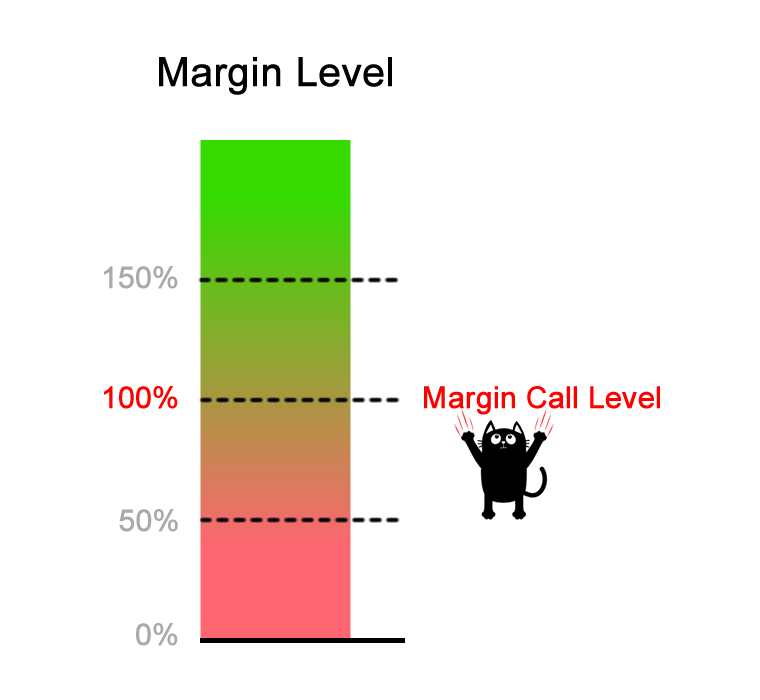## Step 1: Deposit Funds Into Trading Account

Let’s say you have an account balance of \$1,000.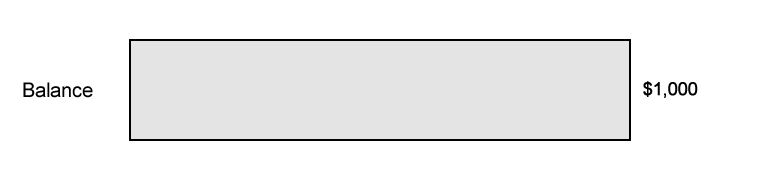Long / Short FX Pair Position Size Entry Price Current Price Margin Level Equity Used Margin Free Margin Balance Floating P/L – \$1,000 – \$1,000 \$1,000 –

## Step 2: Calculate Required Margin

You want to go long EUR/USD at 1.15000 and want to open a 1 mini lot (10,000 units) position. The Margin Requirement is 2%.

How much margin (Required Margin) will you need to open the position?

Since EUR is the base currency. this mini lot is 10,000 euros, which means the position’s Notional Value is €10,000.

Since our trading account is denominated in USD, we need to convert the value of the EUR to USD to determine the Notional Value of the trade.

\$1.15 = €1

\$11,500 = €10,000

The Notional Value is \$11,500.

Now we can calculate the Required Margin:

Required Margin = Notional Value x Margin Requirement

\$230 = \$11,500 x .02

Assuming your trading account is denominated in USD, since the Margin Requirement is 2%, the Required Margin will be \$230.

## Step 3: Calculate Used Margin

Aside from the trade we just entered, there aren’t any other trades open.

Since we just have a SINGLE position open, the Used Margin will be the same as Required Margin.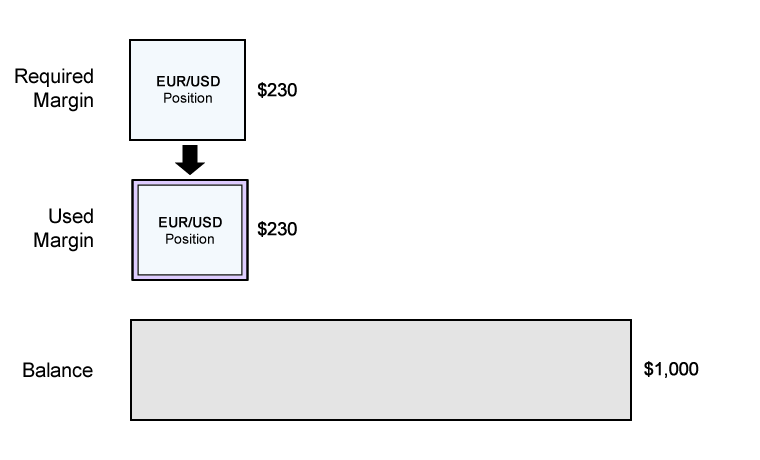## Step 4: Calculate Equity

Let’s assume that the price has moved slightly in your favor and your position is now trading at breakeven.

This means that your Floating P/L is \$0.

Equity = Balance + Floating Profits (or Losses)

\$1,000 = \$1,000 + \$0

The Equity in your account is now \$1,000.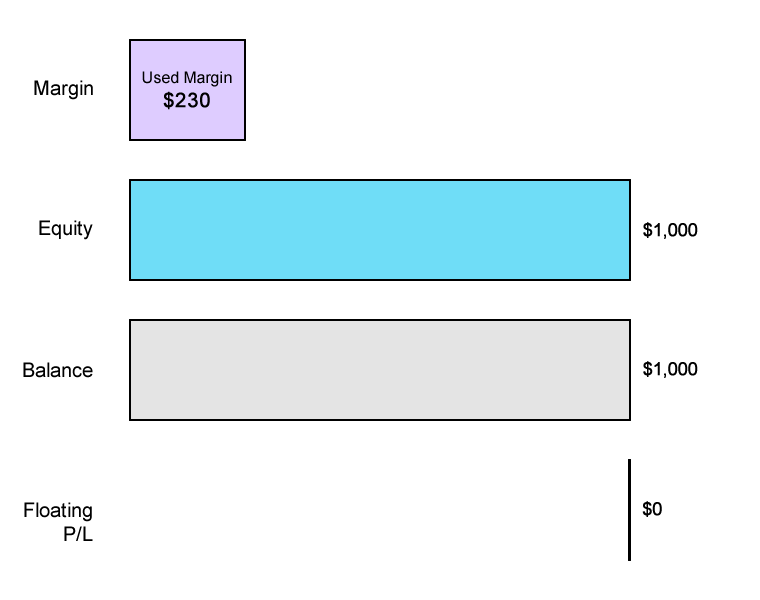## Step 5: Calculate Free Margin

Now that we know the Equity, we can now calculate the Free Margin:

Free Margin = Equity - Used Margin

\$770 = \$1,000 - \$230

## Step 6: Calculate Margin Level

Now that we know the Equity, we can now calculate the Margin Level:

Margin Level = (Equity / Used Margin) x 100%

435% = (\$1,000 / \$230) x 100%

 Long / Short FX Pair Position Size Entry Price Current Price Margin Level Equity Used Margin Free Margin Balance Floating P/L – \$1,000 – – \$1,000 – Long EUR/USD 10,000 1.15000 1.15000 435% \$1,000 \$230 \$770 \$1,000 \$0

## EUR/USD drops 500 pips!There are reports of a zombie outbreak in Paris.

EUR/USD falls 500 pips and is now trading at 1.10000.

Let’s see how your account is affected.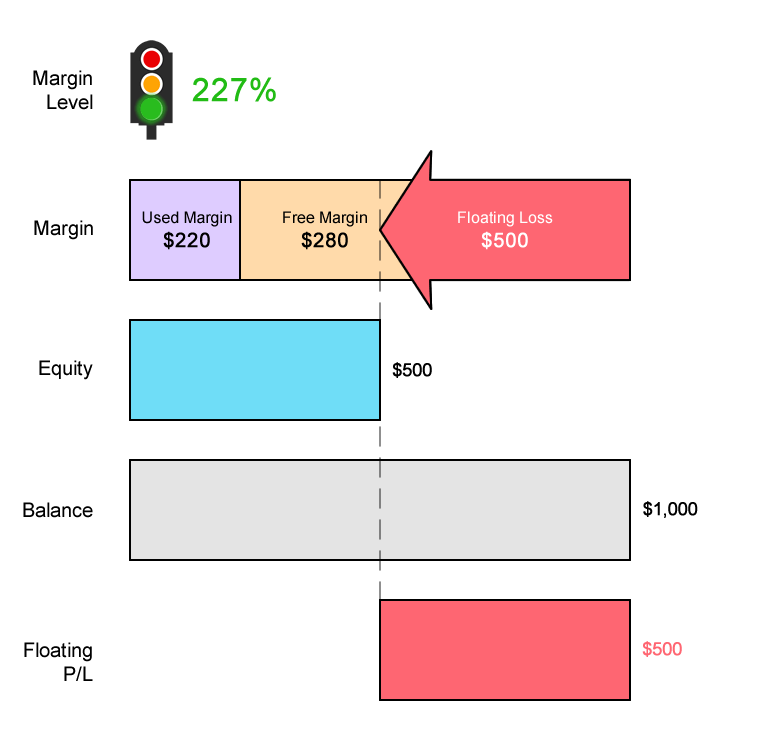### Used Margin

You’ll notice that the Used Margin has changed.

Because the exchange rate has changed, the Notional Value of the position has changed.

This requires recalculating the Required Margin.

Whenever there’s a change in the price for EUR/USD, the Required Margin changes.

With EUR/USD now trading at 1.1000 (instead of 1.15000), let’s see how much Required Margin is needed to keep the position open.

Since our trading account is denominated in USD, we need to convert the value of the EUR to USD to determine the Notional Value of the trade.

\$1.10 = €1

\$11,000 = €10,000

The Notional Value is \$11,000.

Previously, the Notional Value was \$11,500. Since EUR/USD has fallen, this means that EUR has weakened. And since your account is denominated in USD, this causes the position’s Notional Value to decrease.

Now we can calculate the Required Margin:

Required Margin = Notional Value x Margin Requirement

\$220 = \$11,000 x .02

Notice that because the Notional Value has decreased, so has the Required Margin.

Since the Margin Requirement is 2%, the Required Margin will be \$220.

Previously, the Required Margin was \$230 (when EUR/USD was trading at 1.15000).

The Used Margin is updated to reflect changes in Required Margin for every position open.

In this example, since you only have one position open, the Used Margin will be equal to the new Required Margin.

### Floating P/L

EUR/USD has fallen from 1.15000 to 1.10000, a difference of 500 pips.

Since you’re trading 1 mini lot, a 1 pip move equals \$1.

This means that you have a Floating Loss of \$500.

Floating P/L = (Current Price - Entry Price) x 10,000 x \$X/pip

-\$500 = (1.1000 - 1.15000) x 10,000 x \$1/pip

### Equity

Equity = Balance + Floating P/L

\$500 = \$1,000 + (-\$500)

### Free Margin

Your Free Margin is now \$280.

Free Margin = Equity - Used Margin

\$280 = \$500 - \$220

### Margin Level

Your Margin Level has decreased to 227%.

Margin Level = (Equity / Used Margin) x 100%

227% = (\$500 / \$220) x 100%

Your Margin Level is still above 100% so all is still well.

### Account Metrics

 Long / Short FX Pair Position Size Entry Price Current Price Margin Level Equity Used Margin Free Margin Balance Floating P/L – \$1,000 – \$1,000 \$1,000 – Long EUR/USD 10,000 1.15000 1.15000 435% \$1,000 \$230 \$770 \$1,000 \$0 Long EUR/USD 10,000 1.15000 1.10000 227% \$500 \$220 \$280 \$1,000 -\$500

## EUR/USD drops another 288 pips!EUR/USD falls another 288 pips and is now trading at 1.07120.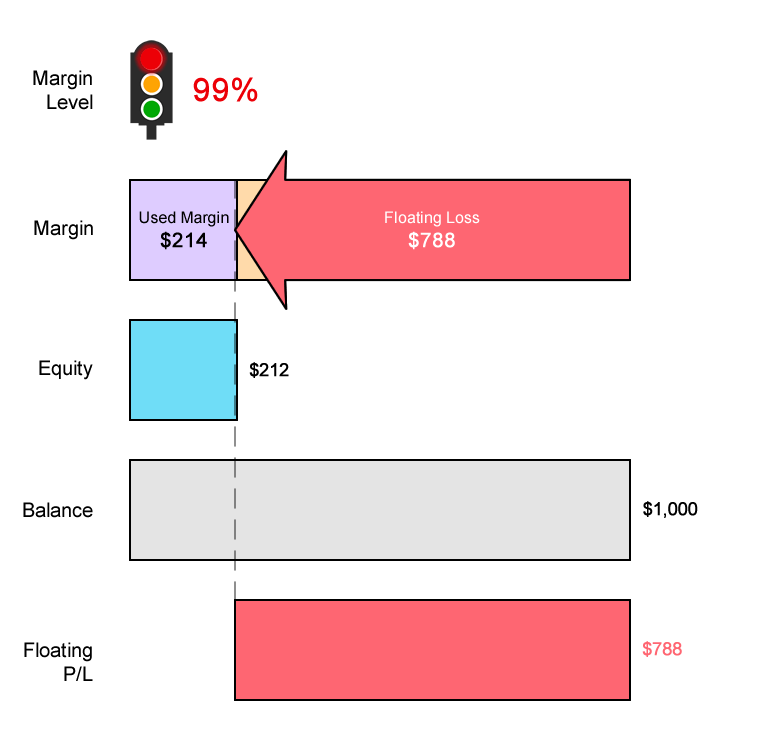### Used Margin

With EUR/USD now trading at 1.07120 (instead of 1.10000), let’s see how much Required Margin is needed to keep the position open.

Since our trading account is denominated in USD, we need to convert the value of the EUR to USD to determine the Notional Value of the trade.

\$1.07120 = €1

\$10,712 = €10,000

The Notional Value is \$10,712.

Now we can calculate the Required Margin:

Required Margin = Notional Value x Margin Requirement

\$214 = \$10,712 x .02

Notice that because the Notional Value has decreased, so has the Required Margin.

Since the Margin Requirement is 2%, the Required Margin will be \$214.

Previously, the Required Margin was \$220 (when EUR/USD was trading at 1.10000).

The Used Margin is updated to reflect changes in Required Margin for every position open.

In this example, since you only have one position open, the Used Margin will be equal to the new Required Margin.

### Floating P/L

EUR/USD has now fallen from 1.15000 to 1.07120, a difference of 788 pips.

Since you’re trading 1 mini lot, a 1 pip move equals \$1.

This means that you have a Floating Loss of \$788.

Floating P/L = (Current Price - Entry Price) x 10,000 x \$X/pip

-\$788 = (1.07120 - 1.15000) x 10,000 x \$1/pip

### Equity

Equity = Balance + Floating P/L

\$212 = \$1,000 + (-\$788)

### Free Margin

Your Free Margin is now –\$2.

Free Margin = Equity - Used Margin

-\$2 = \$212 - \$214

### Margin Level

Your Margin Level has decreased to 99%.

Margin Level = (Equity / Used Margin) x 100%

99% = (\$212 / \$214) x 100%

At this point,  your Margin Level is now below the Margin Call Level!

### Account Metrics

 Long / Short FX Pair Position Size Entry Price Current Price Margin Level Equity Used Margin Free Margin Balance Floating P/L – \$1,000 – \$1,000 \$1,000 – Long EUR/USD 10,000 1.15000 1.15000 435% \$1,000 \$230 \$770 \$1,000 \$0 Long EUR/USD 10,000 1.15000 1.10000 227% \$500 \$220 \$280 \$1,000 -\$500 Long EUR/USD 10,000 1.15000 1.07120 99% \$212 \$214 -\$2 \$1,000 -\$788

## MARGIN CALL!1. Your Used Margin will be “released”.
2. Your Floating Loss will be “realized”.

Your Balance will be updated to reflect the Realized Loss.

Now that your account has no open positions and is “flat”, your Free Margin, Equity, and Balance will be the same.

There is no Margin Level or Floating P/L because there are no open positions.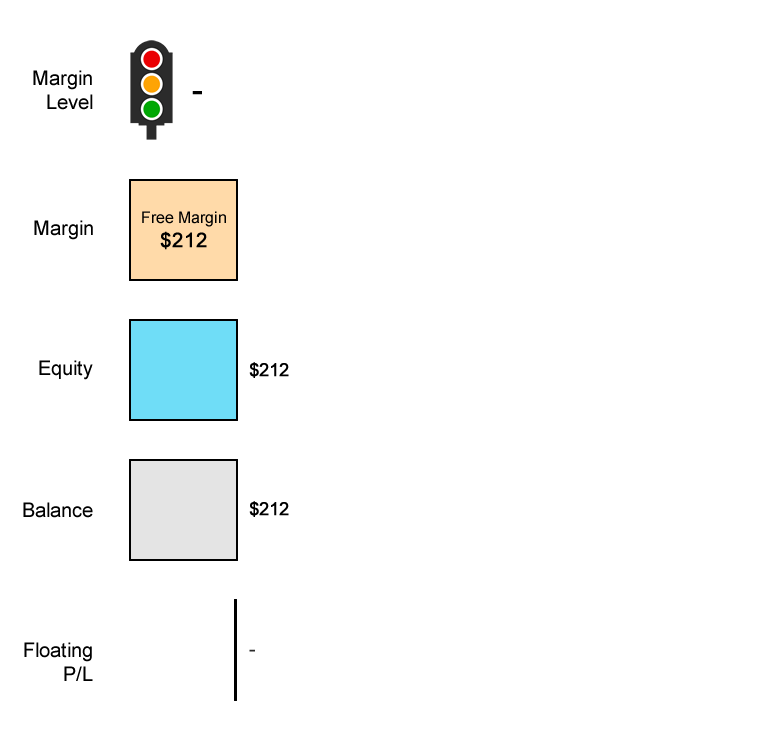Long / Short FX Pair Position Size Entry Price Current Price Margin Level Equity Used Margin Free Margin Balance Floating P/L – \$1,000 – \$1,000 \$1,000 – Long EUR/USD 10,000 1.15000 1.15000 435% \$1,000 \$230 \$770 \$1,000 \$0 Long EUR/USD 10,000 1.15000 1.10000 227% \$500 \$220 \$280 \$1,000 -\$500 Long EUR/USD 10,000 1.15000 1.07120 99% \$212 \$214 -\$2 \$1,000 -\$788 – \$212 – \$212 \$212 –

Before the trade, you had \$1,000 in cash. Now you’re left with \$212!

You’ve lost 79% of your capital.

% Gain/Loss = ((Ending Balance - Starting Balance) / Starting Balance) x 100%

-79% = ((\$212 - \$1,000) / \$1,000) x 100%

Some traders suffer a terrible side effect when finding out their trade has been automatically liquidated.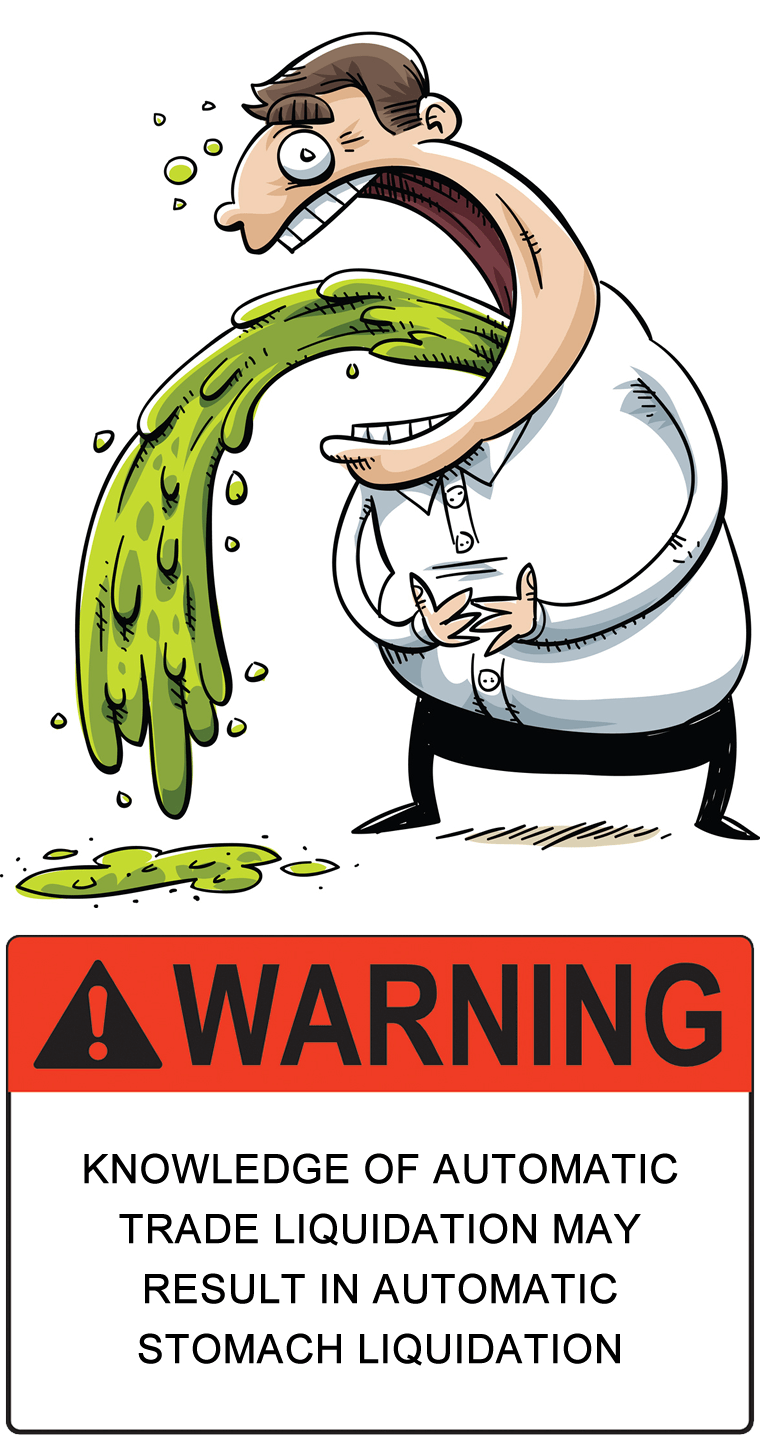In the next lesson, we provide a different trading scenario where your broker has a separate Margin Call AND Stop Out Level.

Let’s see the difference between happens there versus what happened here.WRITTEN BY

## Sebastian TR.

Admin of Traders Resort. Have IT knowledge and great passion in Market.